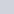Student Forums CMA Part 2 Section E: Investment Decisions E.2. Capital Analysis Analysis Methods Question ID: CMA 695 4.5 (Topic: Net Present Value Method)

# Question ID: CMA 695 4.5 (Topic: Net Present Value Method)

• This topic has 4 replies, 3 voices, and was last updated 9 months, 2 weeks ago byudayca1980.
• Creator
Topic
• #219629udayca1980
Participant

Question:

McLean Inc. is considering the purchase of a new machine that will cost \$160,000. The machine has an estimated useful life of 3 years. Assume that 30% of the depreciable base will be depreciated in the first year, 40% in the second year, and 30% in the third year. The new machine will have a \$10,000 resale value at the end of its estimated useful life. The machine is expected to save the company \$85,000 per year in operating expenses. McLean uses a 40% estimated income tax rate and a 16% hurdle rate to evaluate capital projects.

Discount rates for a 16% rate are as follows:

 Present Value of \$1 Present Value of an Ordinary Annuity of \$1 Year 1 0.862 0.862 Year 2 0.743 1.605 Year 3 0.641 2.246

What is the net present value of this project?

Answer suggested is as follows:

The cash flows are as follows:

 Year 0 Year 1 Year 2 Year 3 Initial Investment (160,000) Depreciation 48,000 64,000 48,000 Depreciation Tax Shield (Depr. × 0.40) 19,200 25,600 19,200 Cash from disposition (after tax) 6,000 Operating cash flows 85,000 85,000 85,000 Tax on operating cash flow at 40% (34,000) (34,000) (34,000) Net Cash Flow 70,200 76,600 76,200 Discount factor: 16% 0.862 0.743 0.641 Discounted Cash Flow (160,000) 60,512 56,914 48,844

The net present value is \$(160,000) + \$60,512 + \$56,914 + \$48,844 = \$6,270

Solution does not take into account the salvage value of \$10k into account (line highlighted in red in above table). I feel that the solution should consider this impact. Could some one advise on this please.

Considering the salvage value in calculation of depreciation shield I get an NPV of \$3,278.

Viewing 4 replies - 1 through 4 (of 4 total)
• Author
Replies
• #219644Brian Hock
HOCK international

The \$10,000 sale of the equipment is in the solution. In the table there is line “Cash from disposition (after tax)” with a cash inflow of \$6,000 in Year 3. This is the \$10,000 gain on the sale reduced by the 40% taxes that they would pay. So, the net effect of the \$10,000 sale price is only \$6,000 for the company.

Does this help?

#219663Lynn Roden
HOCK international

Hi udayca1980,

Are you saying you think the depreciable base should be only \$150,000 because of the salvage value, and therefore the annual depreciation and the cash flow from the annual depreciation tax shield should be lower?

If so, please see the topic in your CMA Part 2, Vol. 2 book, “Depreciation Tax Shield – A Cash Inflow.” It explains there that the amount of depreciation to use in the calculation of net cash flow in capital budgeting must be the depreciation method used for tax purposes. And the method of depreciation used for tax purposes requires that the full cost of an asset be depreciated without regard to any salvage value. Therefore, the annual depreciation to be used in this problem is based on the total cost of \$160,000, not \$160,000 minus \$10,000 for the salvage value.

As a result, the machine is depreciated down to a zero tax basis (the book value according to the tax return) by the end of year 3. Thus, as Brian says, the \$10,000 received from its sale is 100% taxable as a gain, at the 40% tax rate. So the net cash from the sale is \$10,000 x (1 – 0.40), which equals \$6,000.

Lynn

• This reply was modified 9 months, 2 weeks ago byLynn Roden.
#219736udayca1980
Participant

Thanks Roden, clear now.

#219737udayca1980
Participant

Hi Brian,

My question was : Why the residual value is not considered for depreciation purpose. Lynn has answered and I now understand that while for accounting purposes we use the residual value in depreciation calculations, for tax purposes it should not be considered.

Thanks
Uday

Viewing 4 replies - 1 through 4 (of 4 total)
• You must be logged in to reply to this topic.